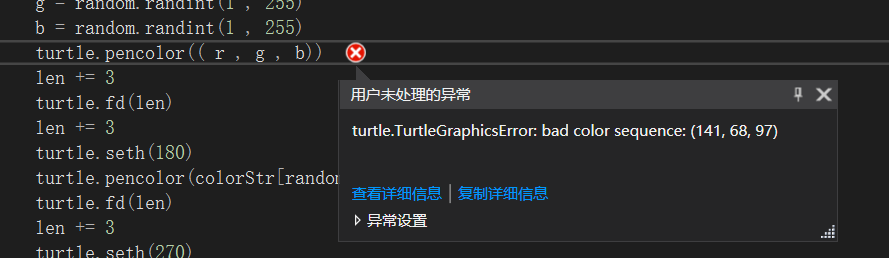python turtle库设置画笔颜色异常

import turtle
import random

#turtle.setup(200,200)
len = 9
colorStr = "darkgreen" ,"purple" , "violet" , "gold"
turtle.pensize(2)
turtle.begin_fill()
for i in range(50):
turtle.seth(90)
r = random.randint(1 , 255)
g = random.randint(1 , 255)
b = random.randint(1 , 255)
turtle.pencolor(( r , g , b))
len += 3
turtle.fd(len)
len += 3
turtle.seth(180)
turtle.pencolor(colorStr[random.randint(0 , 3)])
turtle.fd(len)
len += 3
turtle.seth(270)
turtle.pencolor(colorStr[random.randint(0 , 3)])
turtle.fd(len)
len += 3
turtle.seth(360)
turtle.pencolor(colorStr[random.randint(0 , 3)])
turtle.fd(len)
turtle.end_fill()
turtle.done()2个回答

r,gb必须在colormode内，colormode范围是1.0或255范围，你试试1.0内

r = random.random()
g = random.random()
b = random.random()